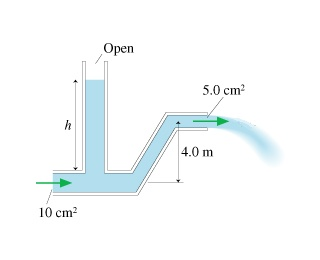# Problem: Water flows from the pipe shown in the figure with a speed of 3.0m/s.What is the height h of the standing column of water?

###### FREE Expert Solution

Equation of continuity:

$\overline{){{\mathbf{A}}}_{{\mathbf{1}}}{{\mathbf{v}}}_{{\mathbf{1}}}{\mathbf{=}}{{\mathbf{A}}}_{{\mathbf{2}}}{{\mathbf{v}}}_{{\mathbf{2}}}}$

Bernoulli's equation:

$\overline{){{\mathbf{P}}}_{{\mathbf{1}}}{\mathbf{+}}\frac{\mathbf{1}}{\mathbf{2}}{\mathbf{\rho }}{{{\mathbf{v}}}_{{\mathbf{1}}}}^{{\mathbf{2}}}{\mathbf{+}}{\mathbf{\rho }}{\mathbf{g}}{{\mathbf{y}}}_{{\mathbf{1}}}{\mathbf{=}}{{\mathbf{P}}}_{{\mathbf{2}}}{\mathbf{+}}\frac{\mathbf{1}}{\mathbf{2}}{\mathbf{\rho }}{{\mathbf{v}}_{\mathbf{2}}}^{{\mathbf{2}}}{\mathbf{+}}{\mathbf{\rho }}{\mathbf{g}}{{\mathbf{y}}}_{{\mathbf{2}}}}$

From the equation of continuity:

v1 = (A2V2)/A1 = [(5.0)(3.0)]/10 = 1.5 m/s

89% (407 ratings)###### Problem DetailsWater flows from the pipe shown in the figure with a speed of 3.0m/s.

What is the height h of the standing column of water?